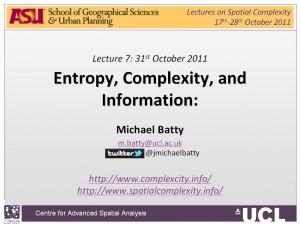# Lecture 7

The seventh lecture takes our third and last form of scaling further with respect to entropy maximising and the interpretation of entropy itself. Basically we review what entropy actually is from raw information, state some general properties and this leads to theShannoninformation H. We then interpret this in terms of the one dimensional population or probability density model showing its extreme distributions from the uniform to the peaked. This defines the range of entropy and we look at an intermediate form – the negative exponential. We show how this distribution can be derived using a random collisions style of model and then we illustrate how we get a power law rather than a negative exponential. We go on to then show how we can change the type of problem to one of city size distributions rather than locational cost and this lets us derive a power law of city sizes which we can switch back to a rank size distribution by transforming it into a counter-cumulative frequency distribution. We then take this entropy further into information differences and then spatial entropy where we define different elements of complexity involving the shape of the distribution, the number of its events, and the areas associated with the events. We conclude with a digression back into spatial interaction delaying with symmetric gravitational models and asymmetric spatial interaction patterns.Here is the lecture. Click on the Full-text PDF size:203 Kb or on the adjacent image. The lecture was first given on Monday 31st October – Halloween – celebrated extensively here but not in class – and posted the same day. Again the material is a little harder than earlier stuff. Read it twice and think about it if you haven’t come across this sort of stuff before.